# Randomized Dictionary Structures:Structural Properties of Skip Lists

###### Structural Properties of Skip Lists

Number of Levels in Skip List

Recall that r = 1 + maxi{Zi}. Notice that Zi k iﬀ the coin tossed for ki gets a run of at least k successes right from the beginning and this happens with probability pk . Since r k iﬀ at least one of Zi k 1 , we easily get the following fact from Boole’s inequality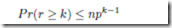Choosing k = 4 log1/p n + 1, we immediately obtain a high conﬁdence bound for the number of levels. In fact,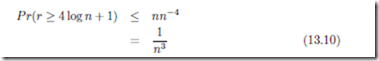We obtain an estimation for the expected value of r, using the formula stated in theorem ( 13.3) as follows: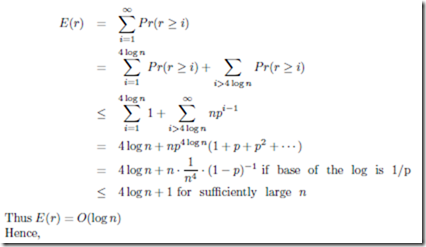THEOREM 13.11 The expected number of levels in a skip list of n elements is O(log n). In fact, the number is O(log n) with high probability.

Space Complexity

Recall that the space complexity, | SL | is given by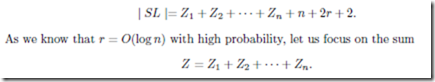Since Zi is a geometric random variable with parameter p, Z is a negative binomial random variable by theorem 13.6.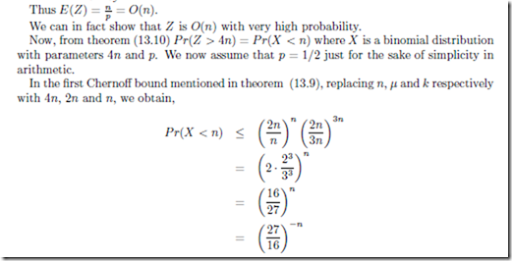This implies that 4n is in fact a very high conﬁdence bound for Z. Since | SL |= Z+n+2r+2, we easily conclude that

THEOREM 13.12 The space complexity of a skip list for a set of size n is O(n) with very high probability.